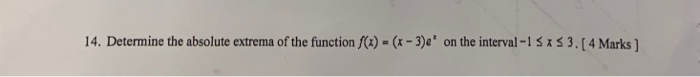# Question Solved1 AnswerDetermine the absolute extrema of the function f(x)=(x-3)e^x on the interval 14. Determine the absolute extrema of the function f(x) = (x- 3)e* on the interval -1 SA $3. [4 Marks ) %3DK64ALA The Asker · Calculus Determine the absolute extrema of the function f(x)=(x-3)e^x on the intervalTranscribed Image Text: 14. Determine the absolute extrema of the function f(x) = (x- 3)e* on the interval -1 SA$ 3. [4 Marks ) %3D
More
Transcribed Image Text: 14. Determine the absolute extrema of the function f(x) = (x- 3)e* on the interval -1 SA \$ 3. [4 Marks ) %3D